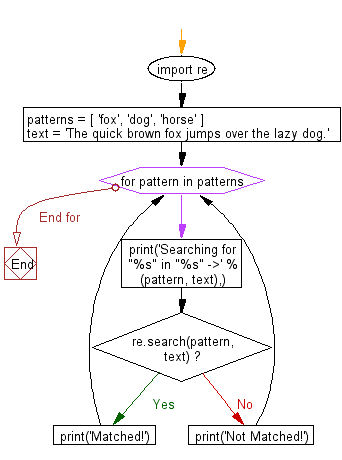﻿ Python Exercises: Search some literals strings in a string - w3resource# Python: Search some literals strings in a string

## Python Regular Expression: Exercise-19 with Solution

Write a Python program to search some literals strings in a string.

Sample Solution:-

Python Code:

``````import re
patterns = [ 'fox', 'dog', 'horse' ]
text = 'The quick brown fox jumps over the lazy dog.'
for pattern in patterns:
print('Searching for "%s" in "%s" ->' % (pattern, text),)
if re.search(pattern,  text):
print('Matched!')
else:
print('Not Matched!')
```
```

Sample Output:

```Searching for "fox" in "The quick brown fox jumps over the lazy dog." ->
Matched!
Searching for "dog" in "The quick brown fox jumps over the lazy dog." ->
Matched!
Searching for "horse" in "The quick brown fox jumps over the lazy dog." ->
Not Matched!
```

Pictorial Presentation:Flowchart:## Visualize Python code execution:

The following tool visualize what the computer is doing step-by-step as it executes the said program:

Python Code Editor:

Have another way to solve this solution? Contribute your code (and comments) through Disqus.

What is the difficulty level of this exercise?

Test your Python skills with w3resource's quiz

﻿

## Python: Tips of the Day

Merges two or more lists into a list of lists, combining elements from each of the input lists based on their positions

Example:

```def tips_merge(*args, fill_value=None):
max_length = max([len(lst) for lst in args])
result = []
for i in range(max_length):
result.append([
args[k][i] if i < len(args[k])
else fill_value for k in range(len(args))
])
return result
print(tips_merge(['x', 'y'], [1, 2], [True, False]))
print(tips_merge(['x'], [1, 2], [True, False]))
print(tips_merge(['x'], [1, 2], [True, False], fill_value = '_'))
```

Output:

```[['x', 1, True], ['y', 2, False]]
[['x', 1, True], [None, 2, False]]
[['x', 1, True], ['_', 2, False]]
```